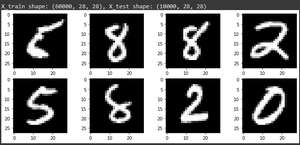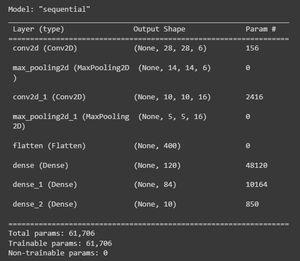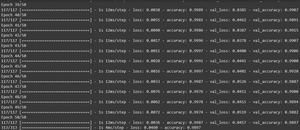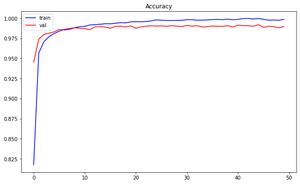# Convolutional Neural Network (CNN) in Tensorflow

• Difficulty Level : Easy
• Last Updated : 02 Mar, 2022

It is assumed that the reader knows the concepts of Neural Networks and Convolutional Neural Networks. If you are sure about the topics please refer to Neural Networks and Convolutional Neural Networks.

### Building Blocks of CNN:

Convolutional Neural Networks are mainly made up of three types of layers:

• Convolutional Layer: It is the main building block of a CNN. It inputs a feature map or input image consisting of a certain height, width, and channels and transforms it into a new feature map by applying a convolution operation. The transformed feature map consists of different height, width, and channels based on the filter_size, padding, and stride.

## Python3

 `import` `tensorflow as tf`` ` `conv_layer ``=` `tf.keras.layers.Conv2D(``    ``filters, kernel_size, strides``=``(``1``, ``1``), padding``=``'valid'``,``    ``data_format``=``None``, dilation_rate``=``(``1``, ``1``), groups``=``1``, activation``=``None``,``    ``use_bias``=``True``, kernel_initializer``=``'glorot_uniform'``,``    ``bias_initializer``=``'zeros'``, kernel_regularizer``=``None``,``    ``bias_regularizer``=``None``, activity_regularizer``=``None``, kernel_constraint``=``None``,``    ``bias_constraint``=``None``, ``*``*``kwargs``)`

• Pooling Layer: Pooling layers do the operation of downsampling, where it reduces the size of the input feature map. Two main types of pooling are Max Pooling and Average Pooling.

## Python3

 `import` `tensorflow as tf`` ` `max_pooling_layer ``=` `tf.keras.layers.MaxPool2D(``    ``pool_size``=``(``2``, ``2``), strides``=``None``, padding``=``'valid'``, data_format``=``None``,``    ``*``*``kwargs``)`` ` `avg_pooling_layer ``=` `tf.keras.layers.AveragePooling2D(``    ``pool_size``=``(``2``, ``2``), strides``=``None``, padding``=``'valid'``, data_format``=``None``,``    ``*``*``kwargs``)`

• Fully-Connected Layer: Each node in the output layer connects directly to a node in the previous layer.

## Python3

 `import` `tensorflow as tf`` ` `fully_connected_layer ``=` `tf.keras.layers.Dense(``    ``units, activation``=``None``, use_bias``=``True``,``    ``kernel_initializer``=``'glorot_uniform'``,``    ``bias_initializer``=``'zeros'``, kernel_regularizer``=``None``,``    ``bias_regularizer``=``None``, activity_regularizer``=``None``, kernel_constraint``=``None``,``    ``bias_constraint``=``None``, ``*``*``kwargs``)`

Here we have seen the basic building blocks of CNN, so now let’s see the implementation of a CNN model in TensorFlow.

### Implementation of LeNet – 5:

LeNet – 5 is a basic convolutional neural network created for the task of handwritten digit recognition for postal codes.

• Loading MNIST Dataset: MNIST Dataset consists of 60,000 train images and 10,000 test images of shape 28 * 28.

## Python3

 `from` `tensorflow.keras.datasets ``import` `mnist``(X_train, y_train), (X_test, y_test) ``=` `mnist.load_data()``print``(f``'X_train shape: {X_train.shape}, X_test shape: {X_test.shape}'``)`` ` `# Plotting random images from dataset`` ` `# import matplotlib.pyplot as plt ``# import random``# plt.figure(figsize = (12,5))``# for i in range(8):``#   ind = random.randint(0, len(X_train))``#   plt.subplot(240+1+i)``#   plt.imshow(X_train[ind], cmap=True)`Plot Random Images

• Preprocessing Data: It includes normalizing the pixel values from 0 to 255 to 0 to 1. The labels for the images are 0 – 9. It is converted into a one-hot vector.

## Python3

 `from` `tensorflow.keras.utils ``import` `to_categorical``   ` `# convert image datatype from integers to floats``X_train ``=` `X_train.astype(``'float32'``)``X_test ``=` `X_test.astype(``'float32'``)``   ` `# normalising piel values``X_train ``=` `X_train``/``255.0``X_test ``=` `X_test``/``255.0``   ` `# reshape images to add channel dimension``X_train ``=` `X_train.reshape(X_train.shape[``0``], X_train.shape[``1``], X_train.shape[``2``], ``1``)``X_test ``=` `X_test.reshape(X_test.shape[``0``], X_test.shape[``1``], X_test.shape[``2``], ``1``)``   ` `# One-hot encoding label ``y_train ``=` `to_categorical(y_train)``y_test ``=` `to_categorical(y_test)`

• Build LeNet-5 model:

## Python3

 `from` `tensorflow.keras.models ``import` `Sequential``from` `tensorflow.keras.layers ``import` `Conv2D, MaxPooling2D, Dense, Flatten`` ` `model ``=` `Sequential()`` ` `# Layer 1``# Conv 1``model.add(Conv2D(filters``=``6``, kernel_size``=``(``5``, ``5``), strides``=``1``, activation ``=` `'relu'``, input_shape ``=` `(``32``,``32``,``1``)))``# Pooling 1``model.add(MaxPooling2D(pool_size``=``(``2``, ``2``), strides ``=` `2``))`` ` `# Layer 2``# Conv 2``model.add(Conv2D(filters``=``16``, kernel_size``=``(``5``, ``5``), strides``=``1``, activation``=``'relu'``))``# Pooling 2``model.add(MaxPooling2D(pool_size ``=` `2``, strides ``=` `2``))`` ` `# Flatten``model.add(Flatten())`` ` `# Layer 3``# Fully connected layer 1``model.add(Dense(units``=``120``, activation``=``'relu'``))`` ` `#Layer 4``#Fully connected layer 2``model.add(Dense(units``=``84``, activation``=``'relu'``))`` ` `#Layer 5``#Output Layer``model.add(Dense(units``=``10``, activation``=``'softmax'``))`` ` `model.``compile``(optimizer``=``'adam'``, loss``=``'categorical_crossentropy'``, metrics``=``[``'accuracy'``])`Model Summary

• Training CNN Model:

## Python3

 `epochs ``=` `50``batch_size ``=` `512``history ``=` `model.fit(X_train, y_train, epochs``=``epochs, batch_size``=``batch_size, ``                    ``steps_per_epoch``=``X_train.shape[``0``]``/``/``batch_size, ``                    ``validation_data``=``(X_test, y_test), ``                    ``validation_steps``=``X_test.shape[``0``]``/``/``batch_size, verbose ``=` `1``)`` ` `_, acc ``=` `model.evaluate(X_test, y_test, verbose ``=` `1``)``print``(``'%.3f'` `%` `(acc ``*` `100.0``))`` ` `plt.figure(figsize``=``(``10``,``6``))``plt.plot(history.history[``'accuracy'``], color ``=` `'blue'``, label ``=` `'train'``)``plt.plot(history.history[``'val_accuracy'``], color ``=` `'red'``, label ``=` `'val'``)``plt.legend()``plt.title(``'Accuracy'``)``plt.show()`Training HistoryEpochs vs Accuracy

Results: LeNet-5 model has given the performance of 98.97% on the MNIST dataset.

The above task was image classification, but using Tensorflow we can perform tasks such as Object Detection, Image Localization, Landmark Detection, Style Transfer.

My Personal Notes arrow_drop_up